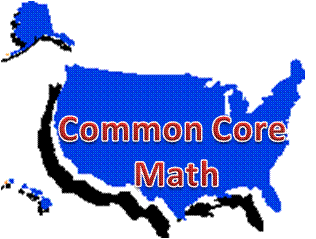# Common Core High School Geometry Teaching and Learning Resources

## Domain: C: Circles• C-A: Understand and apply theorems about circles

• C-B: Find arc lengths and areas of sectors of circles### C-A: Understand and apply theorems about circles

Standards:

• C-A.1. Prove that all circles are similar.
• C-A.2. Identify and describe relationships among inscribed angles, radii, and chords. Include the relationship between central, inscribed, and circumscribed angles; inscribed angles on a diameter are right angles; the radius of a circle is perpendicular to the tangent where the radius intersects the circle.
• C-A.3. Construct the inscribed and circumscribed circles of a triangle, and prove properties of angles for a quadrilateral inscribed in a circle.
• C-A.4. (+) Construct a tangent line from a point outside a given circle to the circle.

Technology-enhanced investigations:

Mathwords.com: Math Dictionary: Key vocabulary for this domain.  Use with C-A and C-B:

 Circle Central Angle Secant Line Sector Radius Inscribed Angle Circumscribed Circle Arc of a Circle Chord Circumscribed Angle* (Geogebra applet) Inscribed Circle Segment of a Circle Diameter Tangent Line Radian

CK-12:Geometry: concepts, videos, and practice exercises for learners to complete: Use with C-A.2.

Khan Academy: Geometric Constructions, Tutorial videos:

LearnZillion:

MathsIsFun.com: Constructions: This is a series of 17 animated geometric constructions, which learners would do with a compass, pencil, and straightedge only.  Among those are circle constructions to find a center, tangent lines from an external point, inscribed and circumscribed circles for triangles, circle touching three points, and a pentagon construction.  Aligns with C-A.3 and C-A.4.

Math Open Reference: Constructions: The site provides step-by-step animations of constructions with compass and straightedge.  The list is comprehensive for lines, line segments, angles, triangles, circles, arcs, ellipses, polygons, and non-Euclidean constructions.  The following align with C-A.3 and C-A.4:

NCTM Illuminations:

• Incenter-Incircle: This applet allows you to find the incenter and incircle of a triangle, and provides a visual explanation of why it occurs where it does.
• Circumcircle: Applet to construct the circumcircle about a triangle.
• Simson Line: Applet to construct and explore the Simson line.

Thinking Mathematics: Tangent Theorems for Circles via Pythagoras: A YouTube video on two results from the geometry of circles that are easily proven using Pythagoras’ theorem.

Wolfram Demonstrations Project: Download the free Wolfram CDF player to interact with the following manipulatives.  Note: Within the Wolfram Demonstration Project are 21 manipulatives addressing C-A.2, 10 manipulatives for C-A.3, and 1 manipulative for C-A.4.  Among those:

Multiple Choice:

MathsIsFun.com: Circle Theorems: Content reviews inscribed angle theorems, angles in quadrilaterals inscribed in circles, the 90 degree angle between a tangent line and the radius drawn to the point of tangency.  Examples are shown.  Learners then complete 10 multiple choice problems on those theorems.  Aligns with C-A.2 and C-A.3.

Constructed-response:

Khan Academy: Geometry: Circles: Practice questions with videos.

Illustrative Mathematics: Geometry:

• Circle and Squares: Students calculate sides of squares given the radius of the inscribed and circumscribed circle and calculate ratios of nested circles and squares.  This task aligns with standards C-A.2 and C-A.3.
• Circles in Triangles: Students use geometric properties of circles and triangles to prove that two triangles are congruent.  This task aligns with geometry congruence standards CO-C.9 and CO-C.10 and geometry circle standards C-A.2 and C-A.3.

Mathematics Assessment Project: High School: Geometry:

Mathematics Vision Project, Secondary 2 Student Edition:

### C-B: Find arc lengths and areas of sectors of circles

Standards:

• C-B.5. Derive using similarity the fact that the length of the arc intercepted by an angle is proportional to the radius, and define the radian measure of the angle as the constant of proportionality; derive the formula for the area of a sector.

Technology-enhanced investigations:

CK-12: Geometry: Arcs in Circles: concepts, videos, and practice problems for learners to complete.

Wolfram Demonstrations Project: Download the free Wolfram CDF player to interact with the following manipulatives.  Note: Within the Wolfram Demonstration Project are 10 manipulatives for C-B.5.  Among those:

Multiple Choice:

MathsIsFun.com: Circle Sector and Segment: Content reviews arc length and area of a sector showing how formulas are derived.  It also includes how to find the area of a segment.  Learners can complete 10 multiple choice problems.  Use with C-B.

Constructed-response:

Khan Academy: Geometry: Circles Practice questions with videos.

• Arc measures in degrees: Aligns with C-B.5.
• Arc Length: Relate the length of the arc to the circumference and the central angle subtended by the arc in degrees.  Aligns with C-B.5.
• Radians and Degrees: Convert angle measures from degrees to radians and vice versa.  Aligns with C-B.5.
• Radians and Arc Length: Solve problems related to radians and arc length, like finding an arc length given a central angle in radians and a radius.
• Area of a sector: Relate the area of a sector to the area of the whole circle.  Aligns with C-B.5.

Illustrative Mathematics: Geometry: Setting up sprinklers: This task aligns with standards in C-B.

Mathematics Assessment Project: High School: Geometry: Task 441: Sectors of Circles

Mathematics Vision Project, Secondary 2 Student Edition: